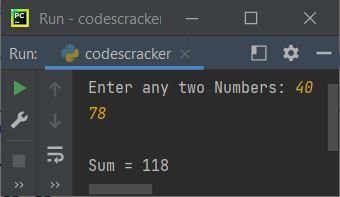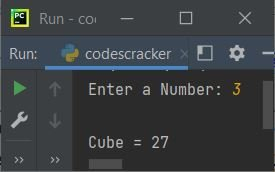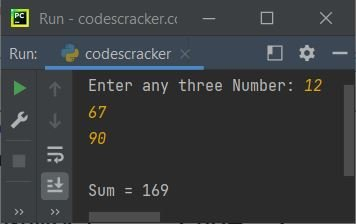# Python lambda Keyword/Function

The lambda keyword is used when we need to create a single expression's anonymous function. For example:

```a = lambda x : x+2
b = lambda x : x*x

print(a(10))
print(b(5))```

The output is:

```12
25```

We can pass any number of arguments to lambda function. For example:

```a = lambda x, y : x+y

print("Enter any two Numbers: ", end="")
numOne = int(input())
numTwo = int(input())

sum = a(numOne, numTwo)
print("\nSum =", sum)```

The snapshot given below shows the sample run of this program, with user input 40 and 78 as two numbers:## Python lambda Function Syntax

The syntax of lambda function in Python, is:

`lambda arg1, arg2, arg3, ..., argN : expression`

The above syntax can also be written in this way:

`lambda arguments : expression`

Where arg1, arg2, and so on, refers to the arguments that will be passed to this function. The return value of this function, will be the result of expression, that comes after evaluating the expression, of course.

## Python lambda Function Example

Let's create some of the examples of lambda function in Python.

### Python lambda Function without Argument

The lambda function can also be created without any arguments. For example:

```hello = lambda: print("Hello World")

hello()```

The output is:

`Hello World`

### Python lambda Function with Single Argument

The program given below is another example of lambda function with single argument:

```cube = lambda x : x*x*x

print("Enter a Number: ", end="")
num = int(input())
print("\nCube =", cube(num))```

The sample run with user input 3, is shown in the snapshot given below:### Python lambda Function with Three Arguments

The following program uses the lambda function to find and return the summation of three numbers:

```sum = lambda a, b, c: a+b+c

print("Enter any three Number: ", end="")
numOne = int(input())
numTwo = int(input())
numThree = int(input())
print("\nSum =", sum(numOne, numTwo, numThree))```

The sample run with user input 12, 67, and 90 as three numbers, is shown in the following snapshot:### Lambda Function as Anonymous Function inside Another Function

Lambda function is called as anonymous function, because it has no name. For example:

```def myfun(n):
return lambda a : a + n + n

x = myfun(2)

print(x(20))```

The output is:

`24`

In above program, the function named myfun with argument value as 2 is initialized to the variable x, using:

`x = myfun(2)`

Therefore, 2 refers to n, for the function myfun(). I've defined a lambda function inside this function, therefore whatever we pass the argument to x, that argument will refer to a of lambda function. Therefore the following code:

`x(20)`

gets evaluated in a way that, 20 refers to a of lambda function. Therefore, the following code:

`lambda a : a + n + n`

gives a + n + n, that is 20 + 2 + 2, that will be 24. And therefore the value 24 is returned using myfun function and printed using the print() statement, of course.

Here is another program, where the lambda function is used as an anonymous function, inside another function:

```def myfun(n):
return lambda a : a + n + n

x = myfun(2)
y = myfun(5)
z = myfun(1)

print(x(20))
print(y(100))
print(z(6))```

The output is:

```24
110
8```

Python Online Test

« Previous Tutorial Next Tutorial »